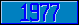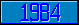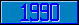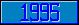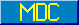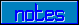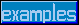## \$%REPLACE

### M[UMPS] by Example

```
2-Jul-96, 6:54:35

Routine Save for Substring Replacement Library function \$%REPLACE^STRING

;

; Unless otherwise noted, the code below

; was approved in document X11/95-112

;

; If corrections have been applied,

; first the original line appears,

; with three semicolons at the beginning of the line.

;

; Then the source of the correction is acknowledged,

; then the corrected line appears, followed by a

; line containing three semicolons.

;

REPLACE(IN,SPEC) ;

SET I="" FOR  SET I=\$ORDER(SPEC(I)) QUIT:I=""  DO

. QUIT:'(\$DATA(SPEC(I,1))#2)

. QUIT:SPEC(I,1)=""

. QUIT:'(\$DATA(SPEC(I,2))#2)

. SET LT=\$LENGTH(SPEC(I,1))

. SET F=0 FOR  SET F=\$FIND(IN,SPEC(I,1),F) QUIT:F<1  DO

. . SET VALUE(F-LT)=SPEC(I,2)

. . QUIT

. QUIT

SET VALUE="" FOR K=1:1:L DO

. IF \$EXTRACT(MASK,K)=" " SET VALUE=VALUE_\$EXTRACT(IN,K) QUIT

. SET:\$DATA(VALUE(K)) VALUE=VALUE_VALUE(K)

. QUIT

QUIT VALUE

;===

;

;

;

```